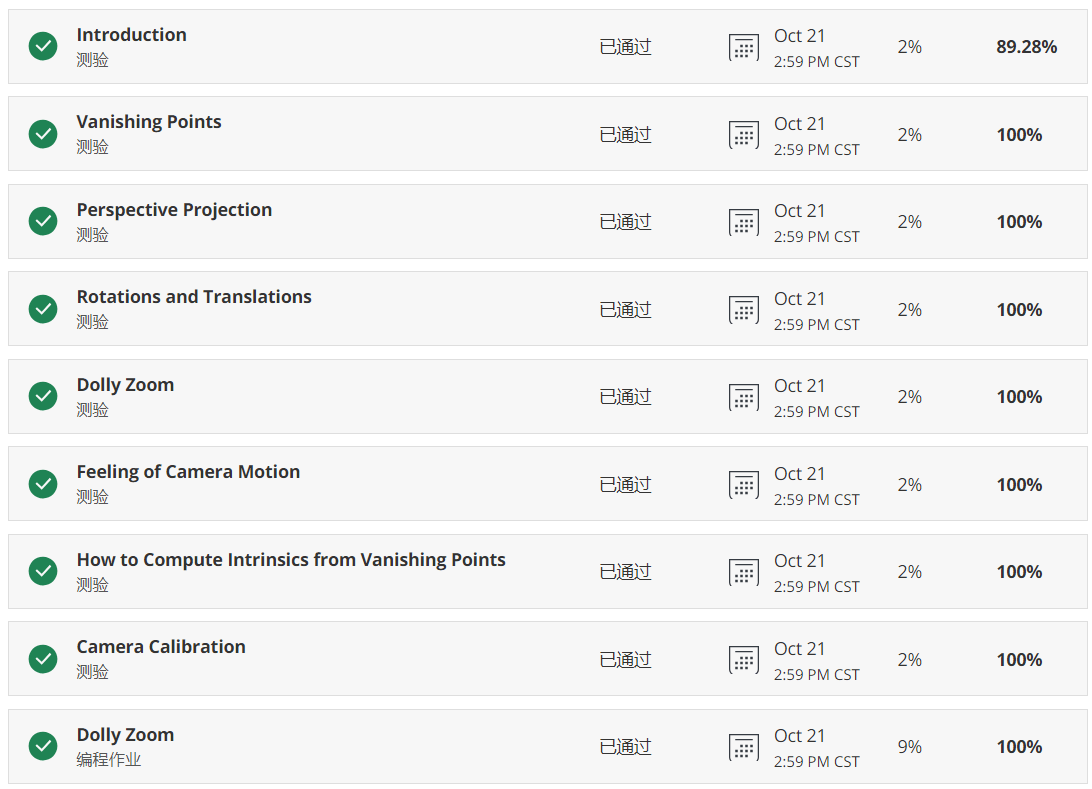# Robotics:Perception课程测验&作业1

## Oct 17,2019   4547 words   17 min

Tags: SLAM

Robotics:Perception课程Week1的测验与作业习题，如果有错误的地方欢迎提出、交流。注意本博客中的题目与答案仅供学习交流使用，严禁用于抄袭等不守信行为。若因抄袭答案导致Coursera账号被取消激活，本博客概不负责。

#### 1.Introduction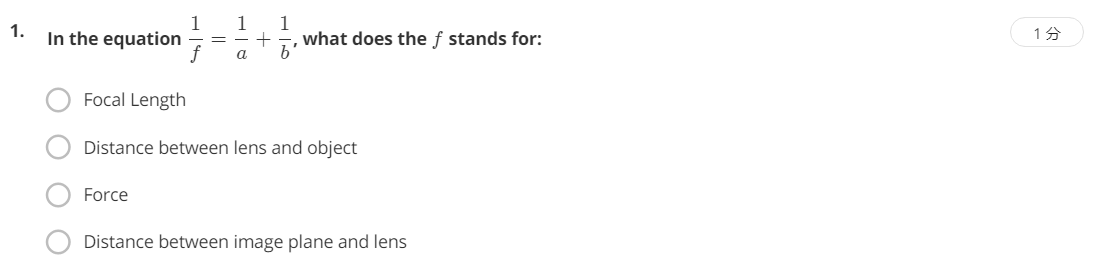考察的是透镜成像模型的成像公式中各变量的含义，f表示焦距，a表示物距，b表示像距，所以选择A，”Focal Length”。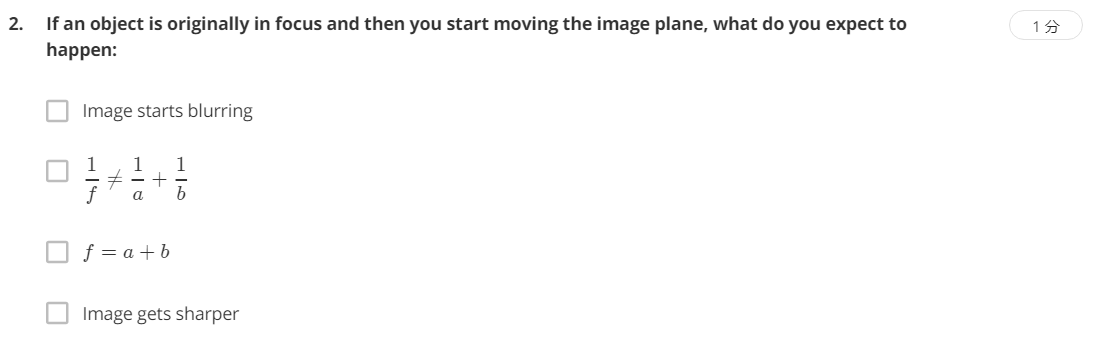考察对焦过程中的变化情况。由于改变了像距b，所以成像公式也不再成立，同时影像开始变得模糊。选择A和B。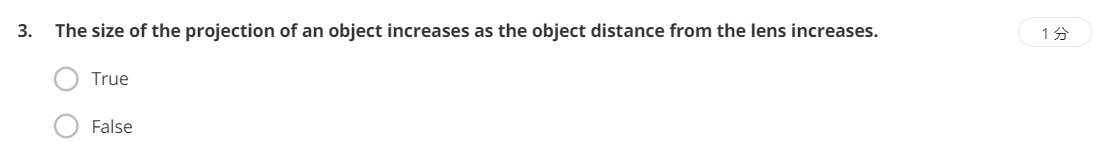考察近大远小的成像规律：

$\frac{Y}{a}=\frac{y}{b}$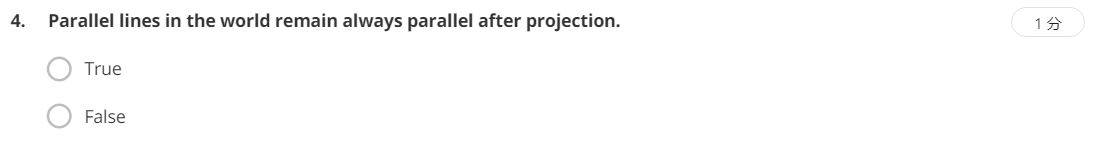False。只有当平行线所在平面与像面平行时，在影像中才会保持平行，否则投影后都不再平行。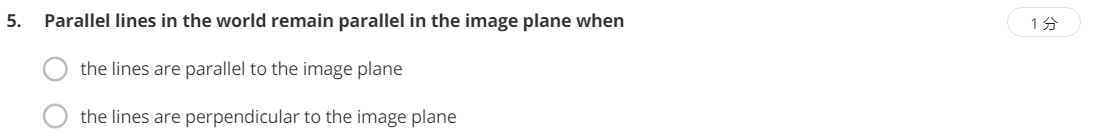和上题一样。只有当线平行于像面时才会保持平行，应选择A。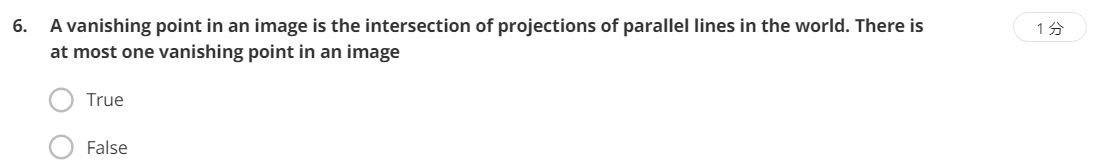False。考察灭点的概念。正如题中所说，灭点是现实世界中一组平行线在像面上的投影线的交点。而在像面中可以有多对现实世界中平行的线，因此也就会有多个交点，这些交点都是灭点。多个灭点连接形成灭影线。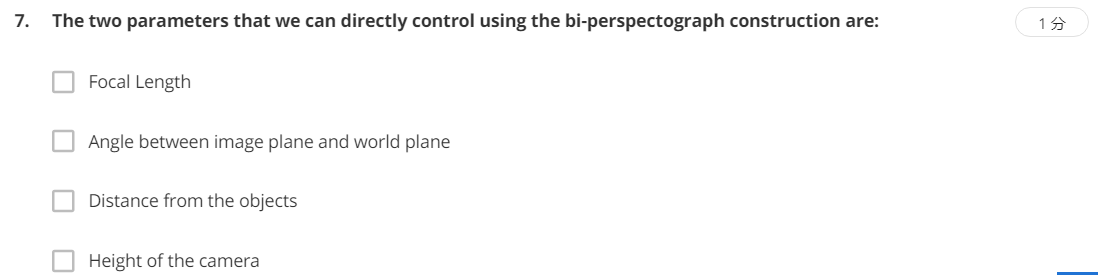答案不全。选B。这个绘图仪的性质。

#### 2.Vanishing Points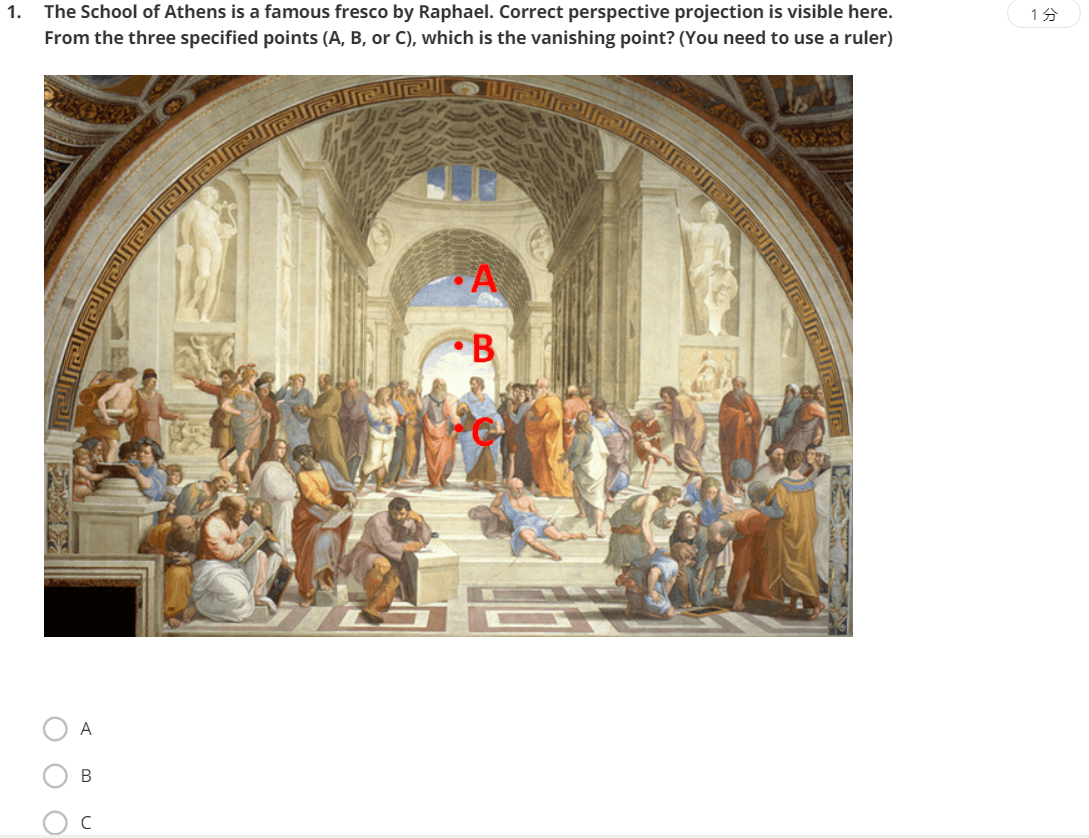考察灭点的定义。很简单，在图像中选取一组现实世界中的平行线，然后找交点即可，可以发现交点是C。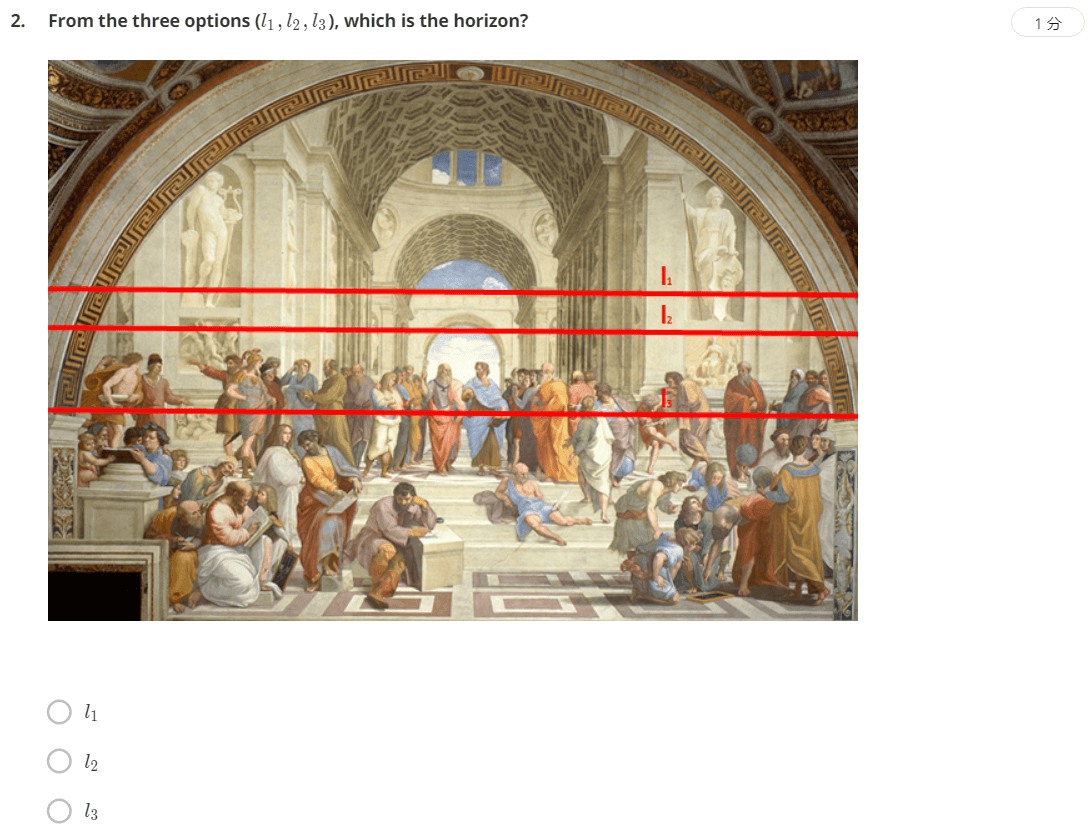和上一题同理，可以找多组平行线，然后这些灭点所在的直线即为地平线。答案为l3。和上一题一样，连接平行线找交点，结果是l1。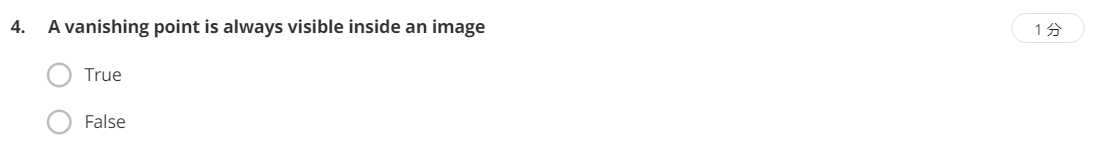很显然是False的。灭点并不一定在影像中，没有必然联系。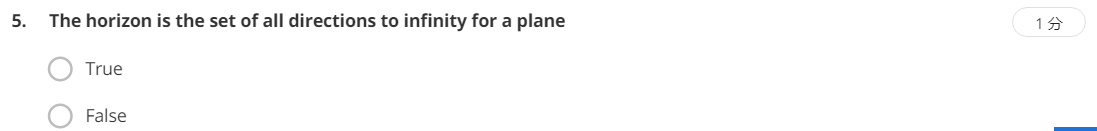True。由灭点组成的灭影线表示无穷远处。

#### 3.Perspective Projection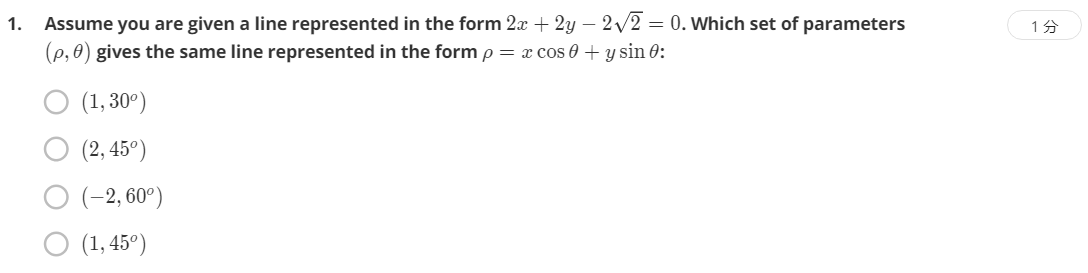由题可知，a=2，b=2，c=-2√2。再根据公式：

$cos\theta = \frac{a}{\sqrt{a^2+b^2}}$ $\rho = \frac{-c}{\sqrt{a^2+b^2}}$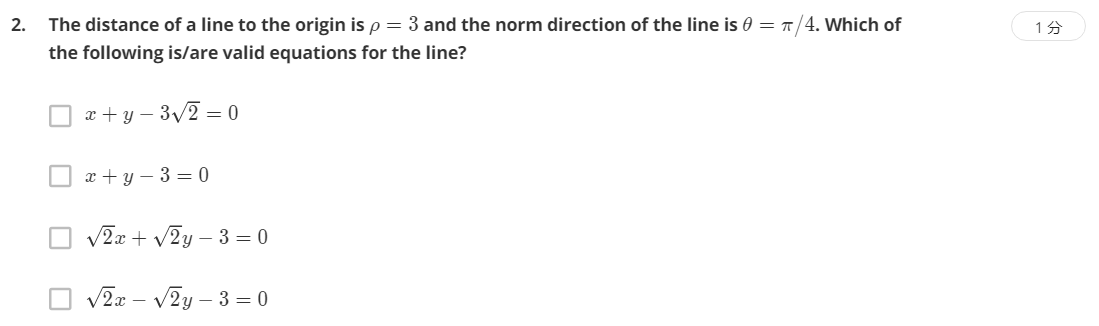还是根据上面的公式，这里θ为45度，ρ为3，将上面式子中的a、b、c依次带入即可算出结果，选A。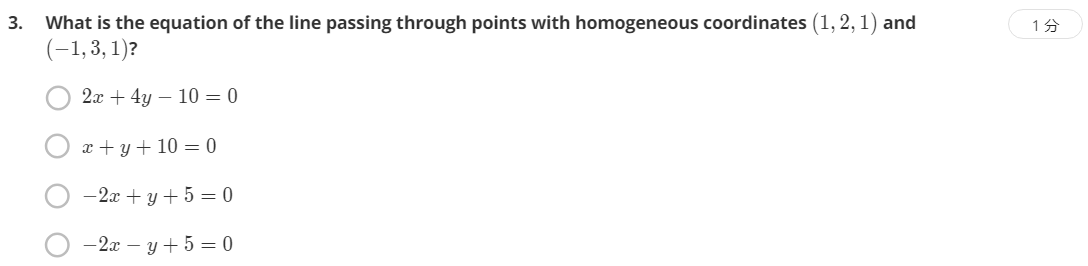考察齐次坐标系下空间直线的表示方法。在齐次坐标系中，直线用其上两个点的向量叉乘得到。所以对(1,2,1)和(-1,3,1)做叉乘，结果为(-1,-2,5)。选项中对应关系正确的是A。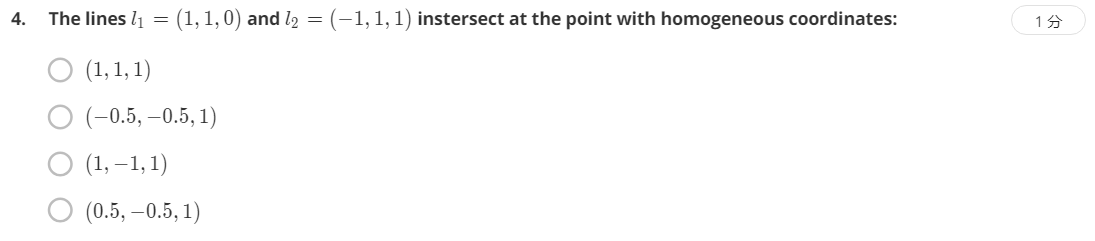齐次坐标系中，求两条直线的交点，用表示两条直线的向量叉乘即可，叉乘结果为(1,-1,2)，对应关系正确的是D。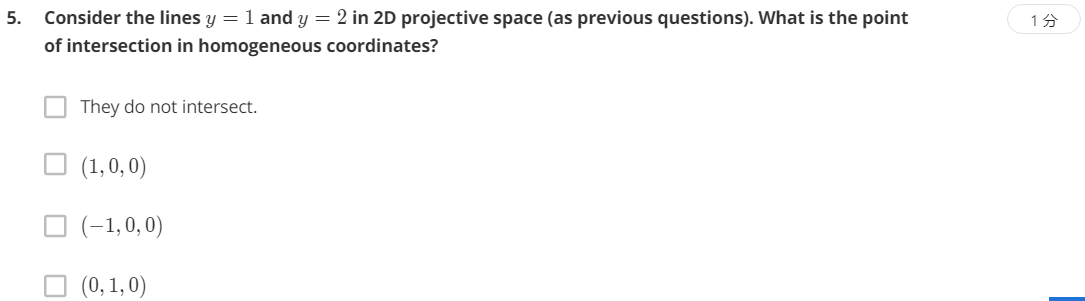考察直线的齐次坐标表示以及直线角点求法。y=1直线用齐次坐标表示，不妨任意取其上的两个点:(0,1,1)、(2,1,1)。这两个点都在z=1的像面上，且满足y=1条件。将这两个向量做叉乘，即可得到y=1的齐次表示为(0,2,-2)。同理y=2可求出为(0,2,-4)。求两条直线的交点做叉乘，得到(-4,0,0)，等价于k(1,0,0)。所以应该选B、C。

#### 4.Rotations and Translations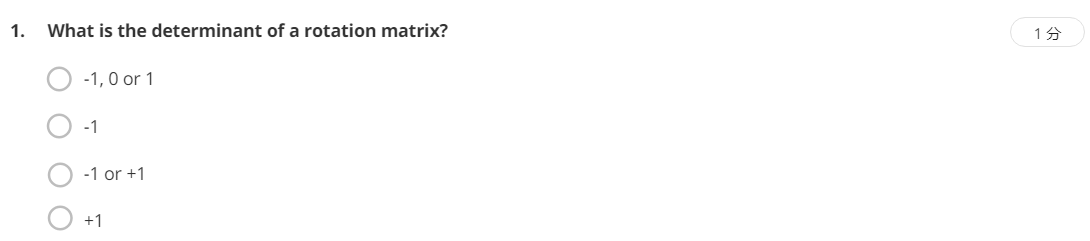考察旋转矩阵的性质。由于旋转矩阵是正交阵，等价于问正交阵的行列式是多少。由正交阵性质：转置等于逆，所以有AA^T=E。对等式两边取行列式，有|AA^T|=|E|，单位阵的行列式值为1，所以|AA^T|=1。又由矩阵行列式的性质：矩阵转置的行列式等于其本身的行列式，所以|A|=|A^T|，所以|A|^2=1，所以|A|的解为正负1。但由于旋转矩阵的特殊性，行列式为-1的情况被舍去，最终答案应该是1，选D。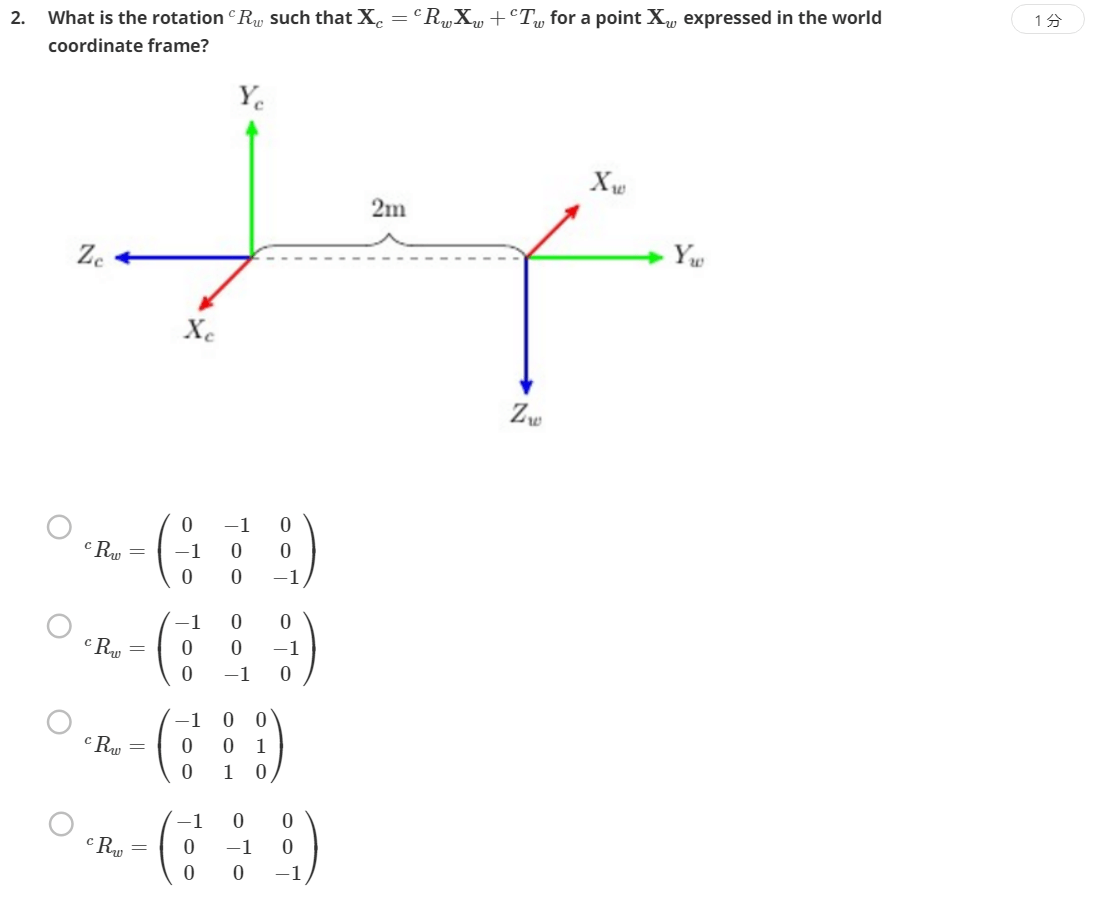考察旋转矩阵的定义。题目中问的是$${}^{c}\textrm{R}_{w}$$，也就是在Camera坐标系下World坐标系的旋转矩阵。World坐标系的x轴在Camera坐标系下表示是(-1,0,0)，y轴表示为(0,0,-1)，z轴表示为(0,-1,0)。所以组合成旋转矩阵应该选B。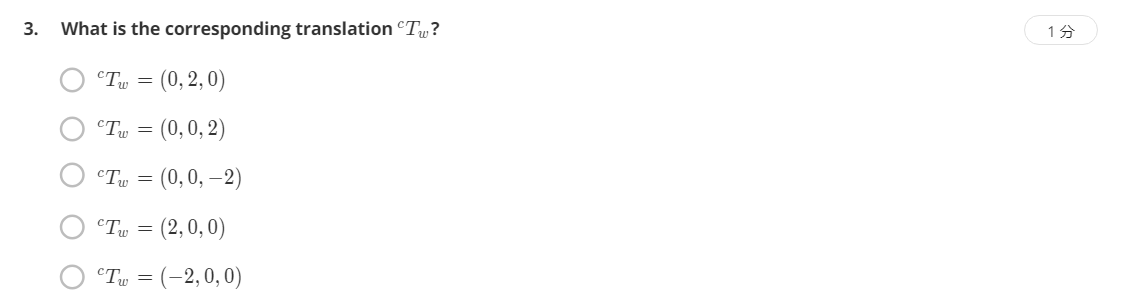同理，同样是考察平移的定义。题目中问的是$${}^{c}\textrm{T}_{w}$$，也就是从Camera到World的平移，由图可知，World坐标系的原点在Camera坐标系中为(0,0,-2)，应选C。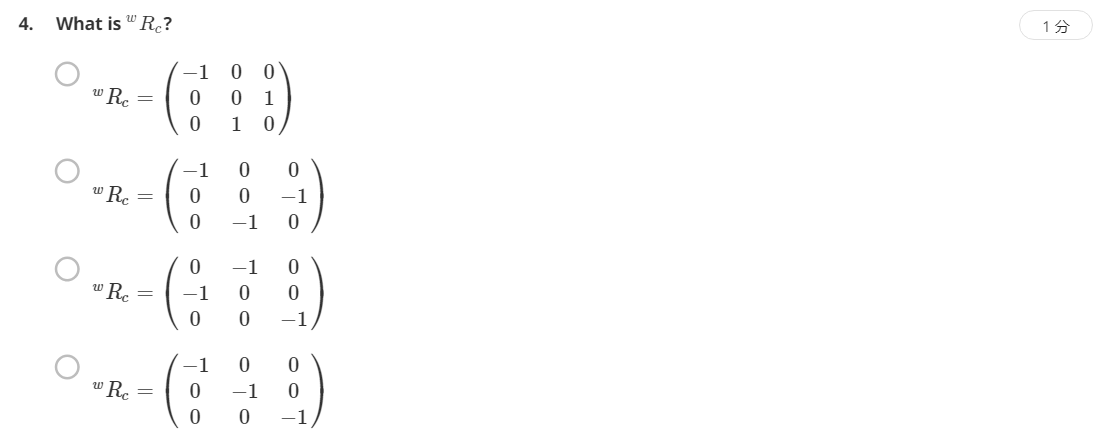注意题目中问的是$${}^{w}\textrm{R}_{c}$$，也就是Camera坐标系相对于World坐标系的旋转，类似的，Camera坐标系的x轴在World坐标系中表示为(-1,0,0)，y轴为(0,0,-1)，z轴为(0,-1,0)，组合成旋转矩阵，应选B。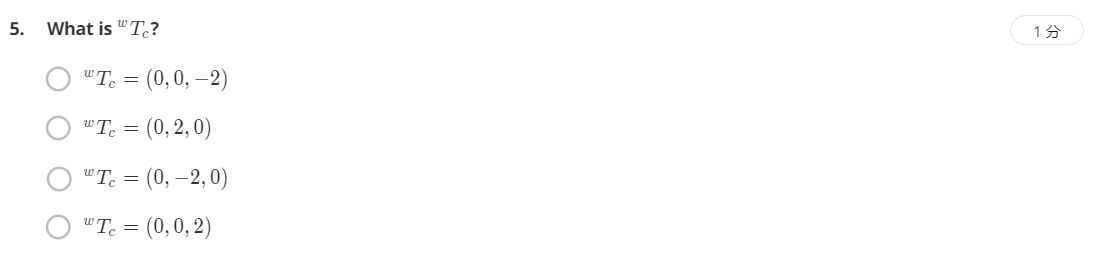同理，题目中问的是Camera坐标系相对于World坐标系的平移，由图可知应为(0,-2,0)，选C。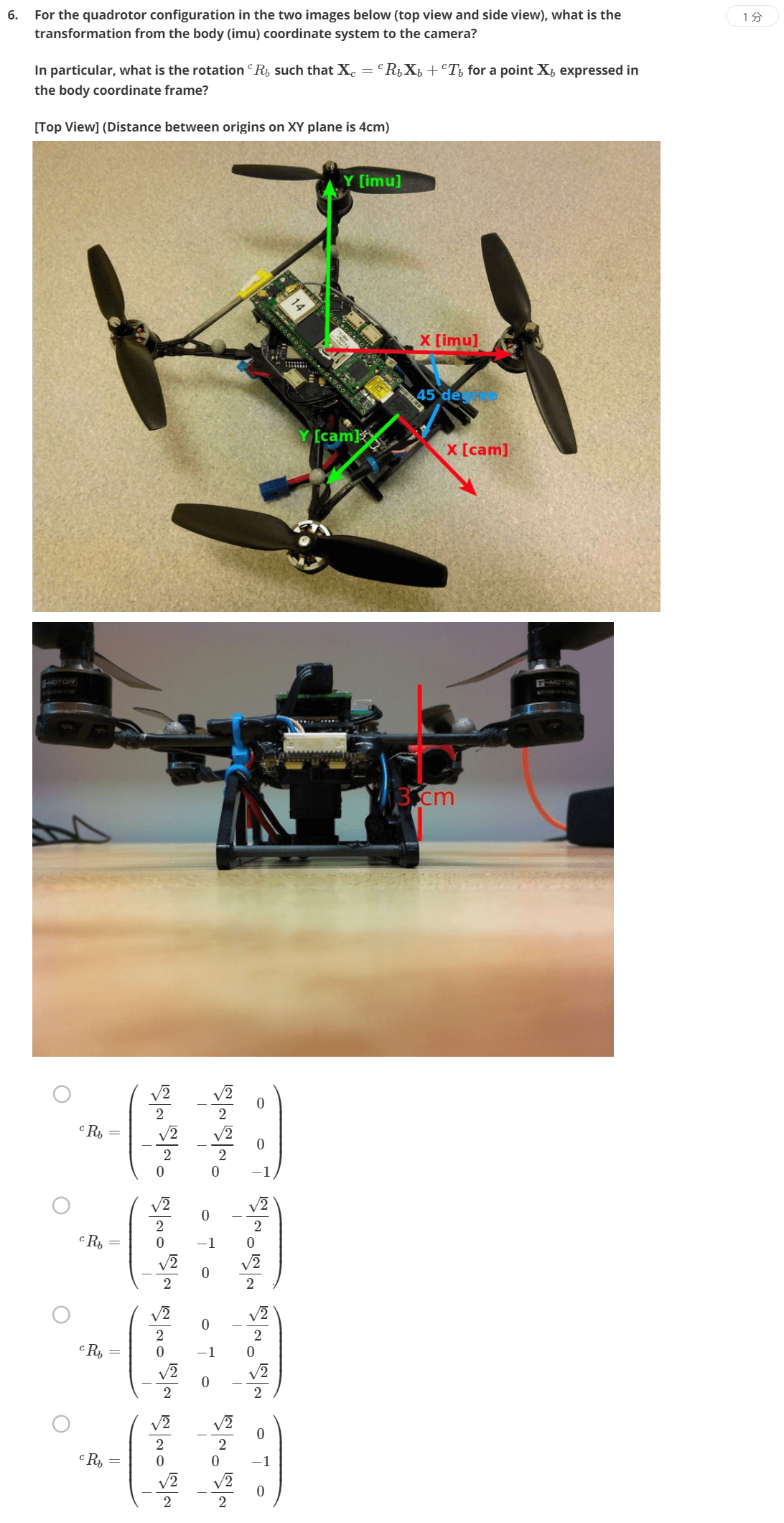看清题目问的是什么，题目问的是Body坐标系相对于Camera坐标系的旋转，两种思路，一是分别找到Body坐标系的x、y、z在Camera坐标系下的表示，然后拼成旋转矩阵；二是直接用旋转合成的方式计算旋转矩阵。

$\begin{pmatrix} \frac{\sqrt{2}}{2} & -\frac{\sqrt{2}}{2} & 0\\ -\frac{\sqrt{2}}{2} & -\frac{\sqrt{2}}{2} & 0\\ 0 & 0 & -1 \end{pmatrix}$

$R_{z,-45}R_{x,180}=\begin{pmatrix} cos(-45) & -sin(-45) & 0\\ sin(-45) & cos(-45) & 0\\ 0 & 0 & 1 \end{pmatrix}\begin{pmatrix} 1 & 0 & 0\\ 0 & cos(180) & -sin(180)\\ 0 & sin(180) & cos(180) \end{pmatrix}$

$\begin{pmatrix} \frac{\sqrt{2}}{2} & -\frac{\sqrt{2}}{2} & 0\\ -\frac{\sqrt{2}}{2} & -\frac{\sqrt{2}}{2} & 0\\ 0 & 0 & -1 \end{pmatrix}$

$R_{x,180}R_{z,45}=\begin{pmatrix} 1 & 0 & 0\\ 0 & cos(180) & -sin(180)\\ 0 & sin(180) & cos(180) \end{pmatrix}\begin{pmatrix} cos(45) & -sin(45) & 0\\ sin(45) & cos(45) & 0\\ 0 & 0 & 1 \end{pmatrix}$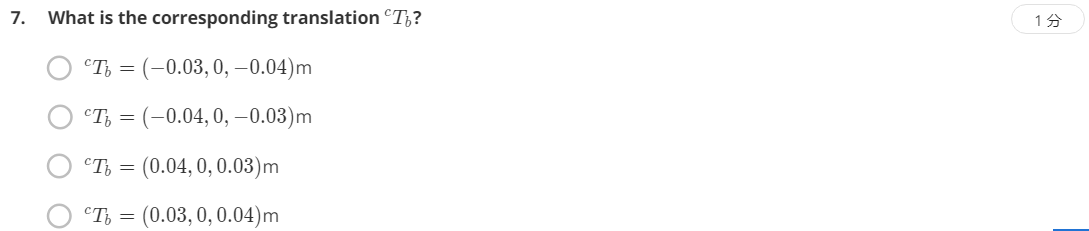题目问的是Camera坐标系下Body的平移，由图可知，Body坐标系沿Camera坐标系x轴负向移动了4cm，沿z轴负向移动了3cm(注意Camera坐标系的z轴正向是向下的)。所以答案为(-0.04,0.-0.03)，应选B。

#### 5.Dolly Zoom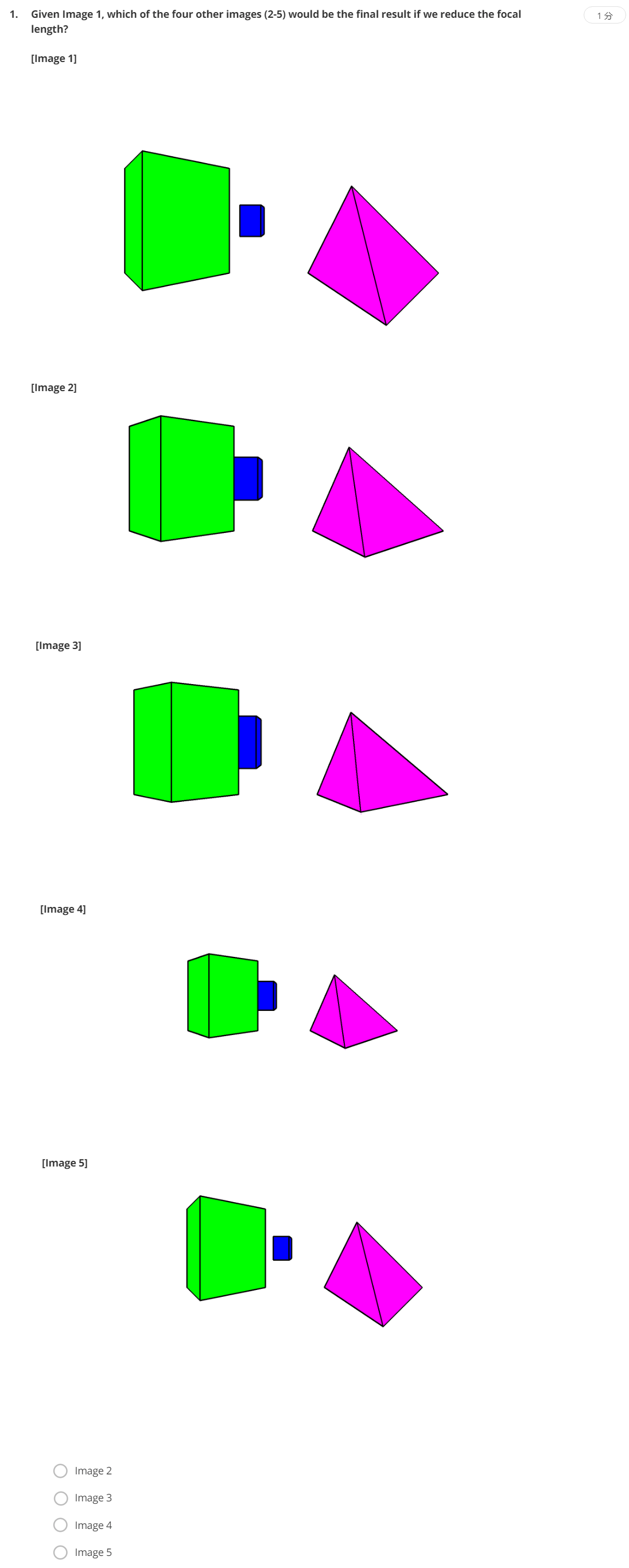考察对Dolly Zoom效果的理解，保持前景物体不变，背景物体随着焦距的增加以及相机的远离，背景物体逐渐变大。但题目中说的是以第一张图片为参考，减小焦距的结果。随着相机逐渐远离，物体会慢慢变小，而减小焦距，根据成像大小公式，也会导致物体变小。所以应该选Image5，E。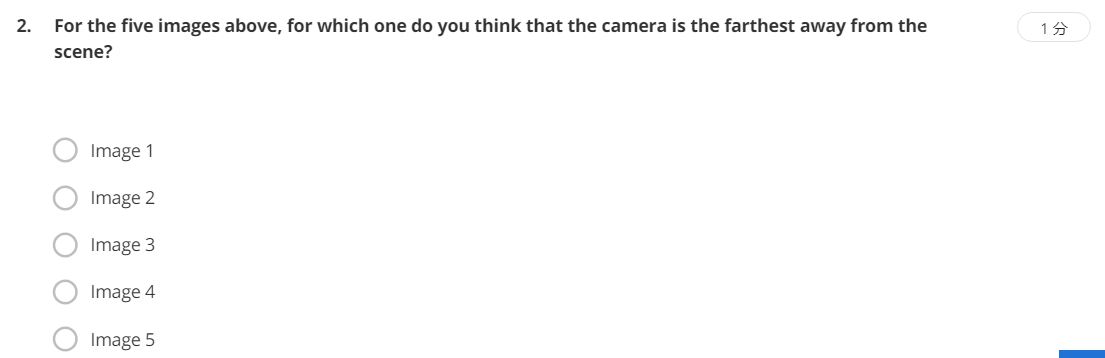和上一题类似，应该选Image3，C。

#### 6.Feeling of Camera Motion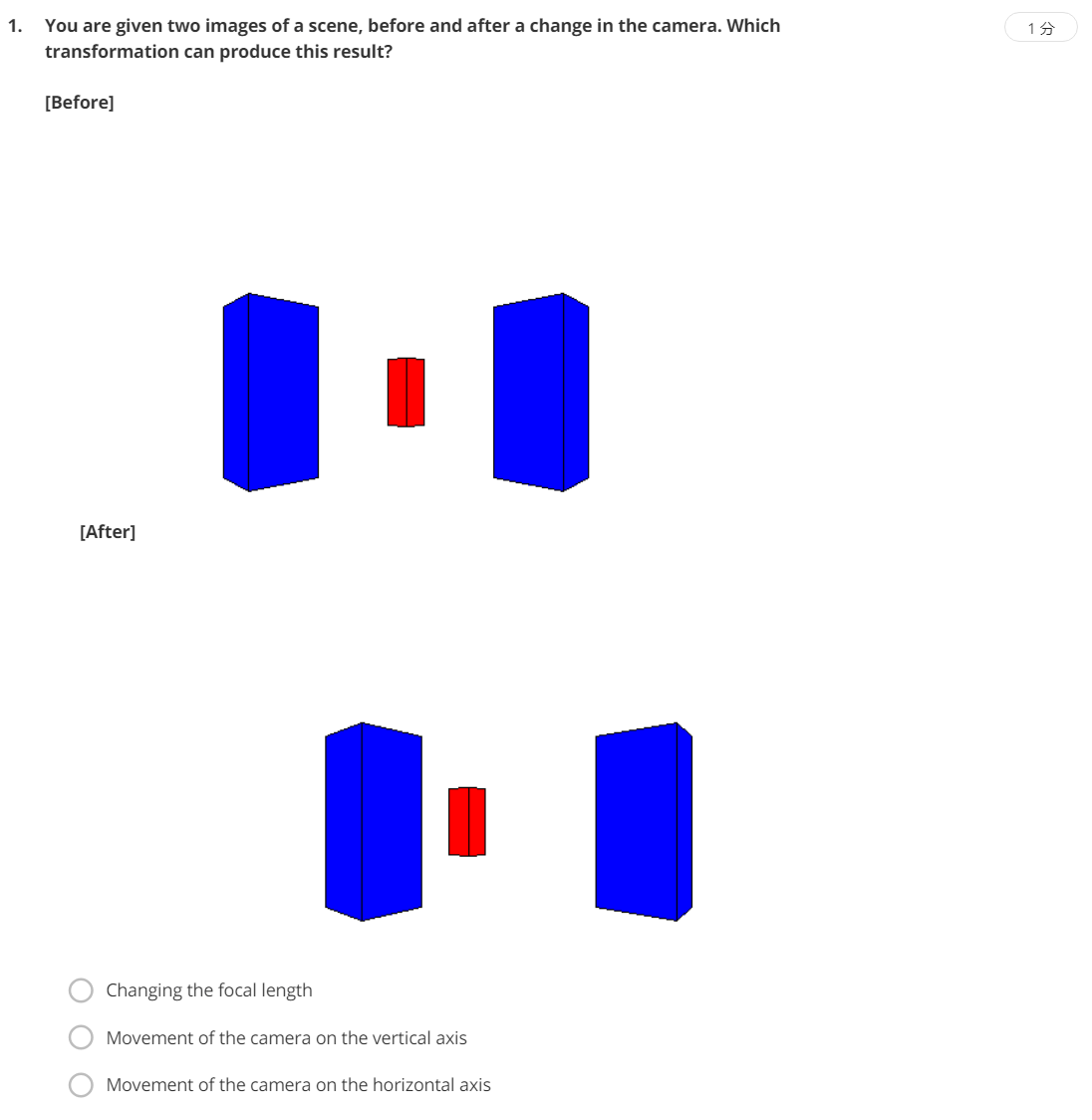这题很明显是相机在水平方向上有运动，所以选C。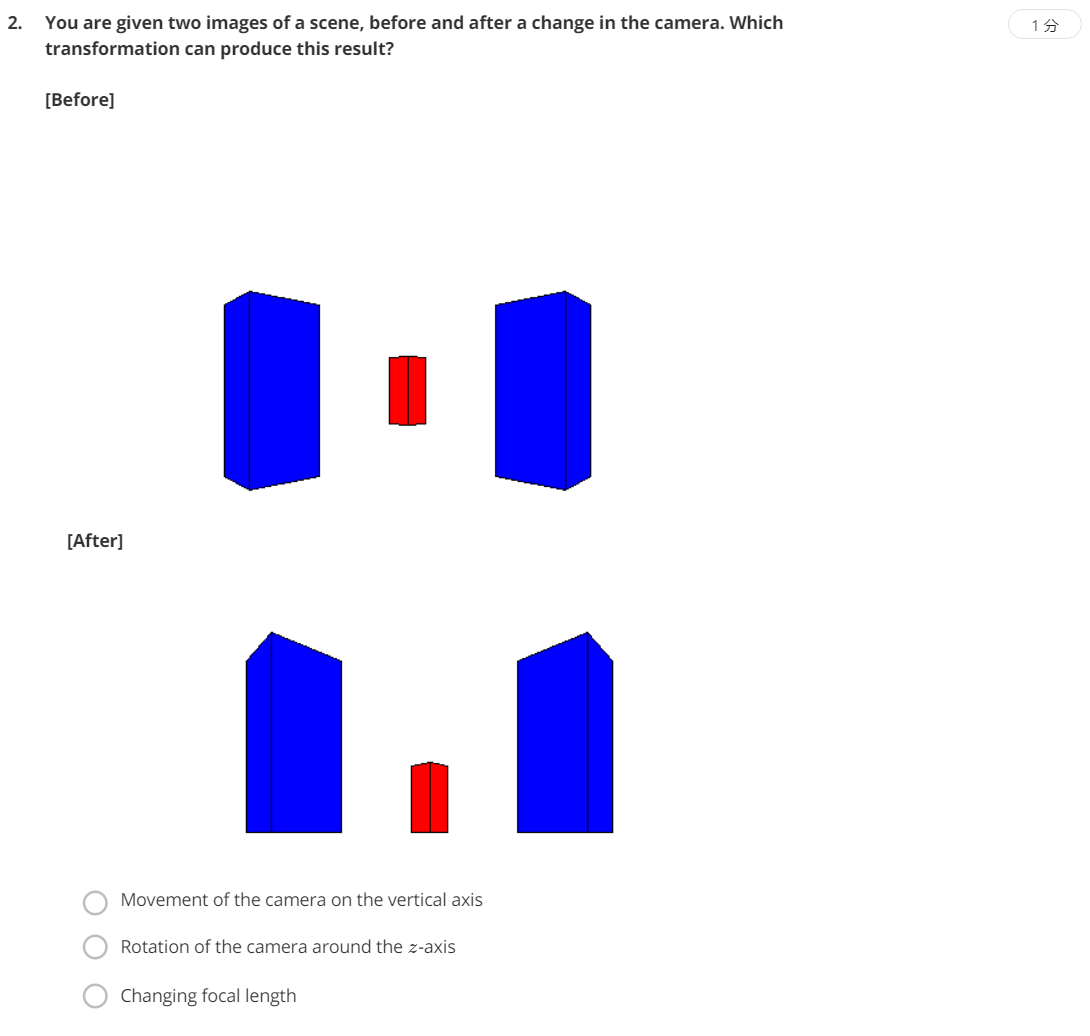这题很明显是相机在竖直方向上有运动，所以选A。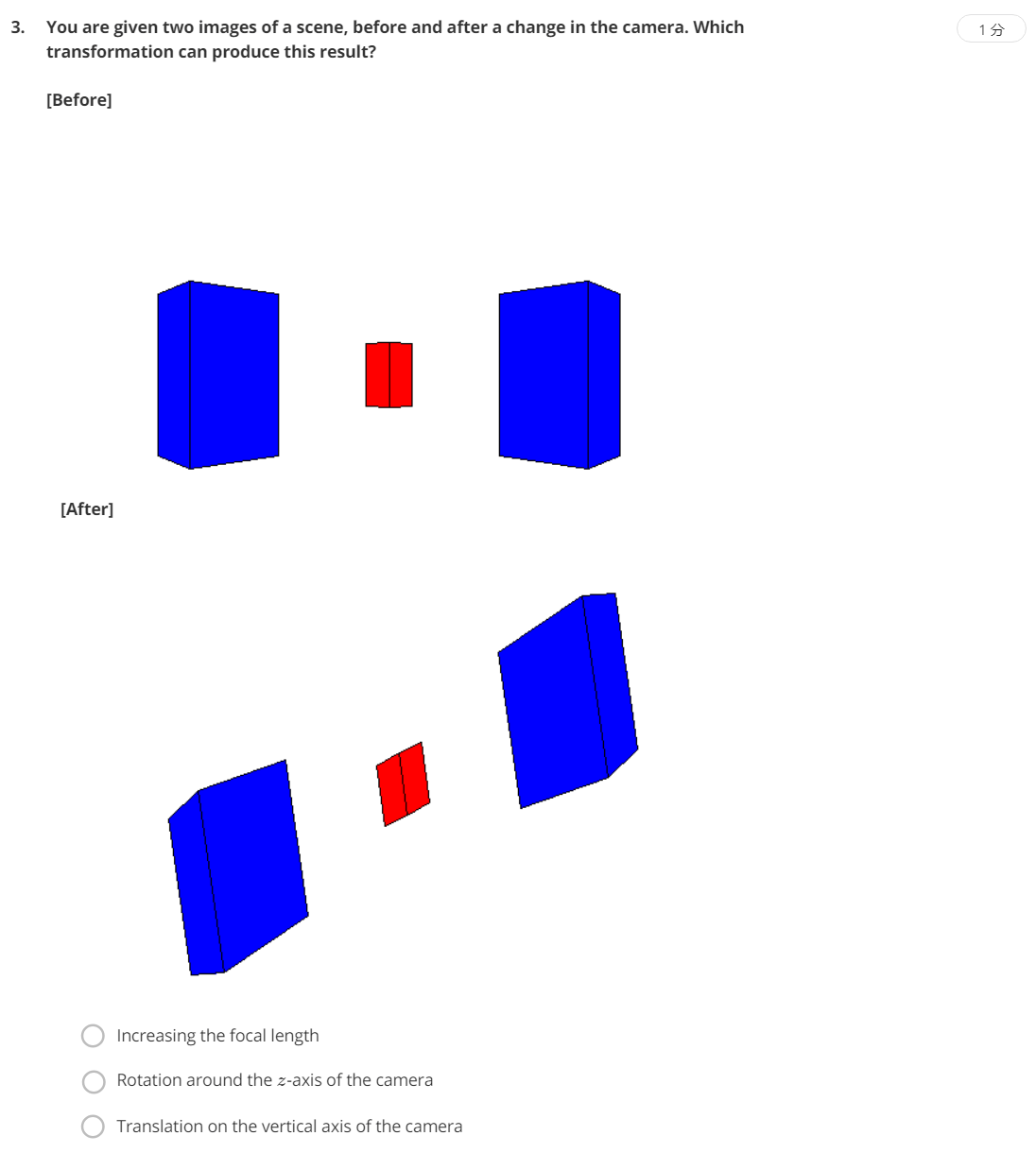图片中的物体向左旋转，相机绕z轴向右旋转。如果不好理解可以用手机试试。

#### 7.How to Compute Intrinsics from Vanishing Points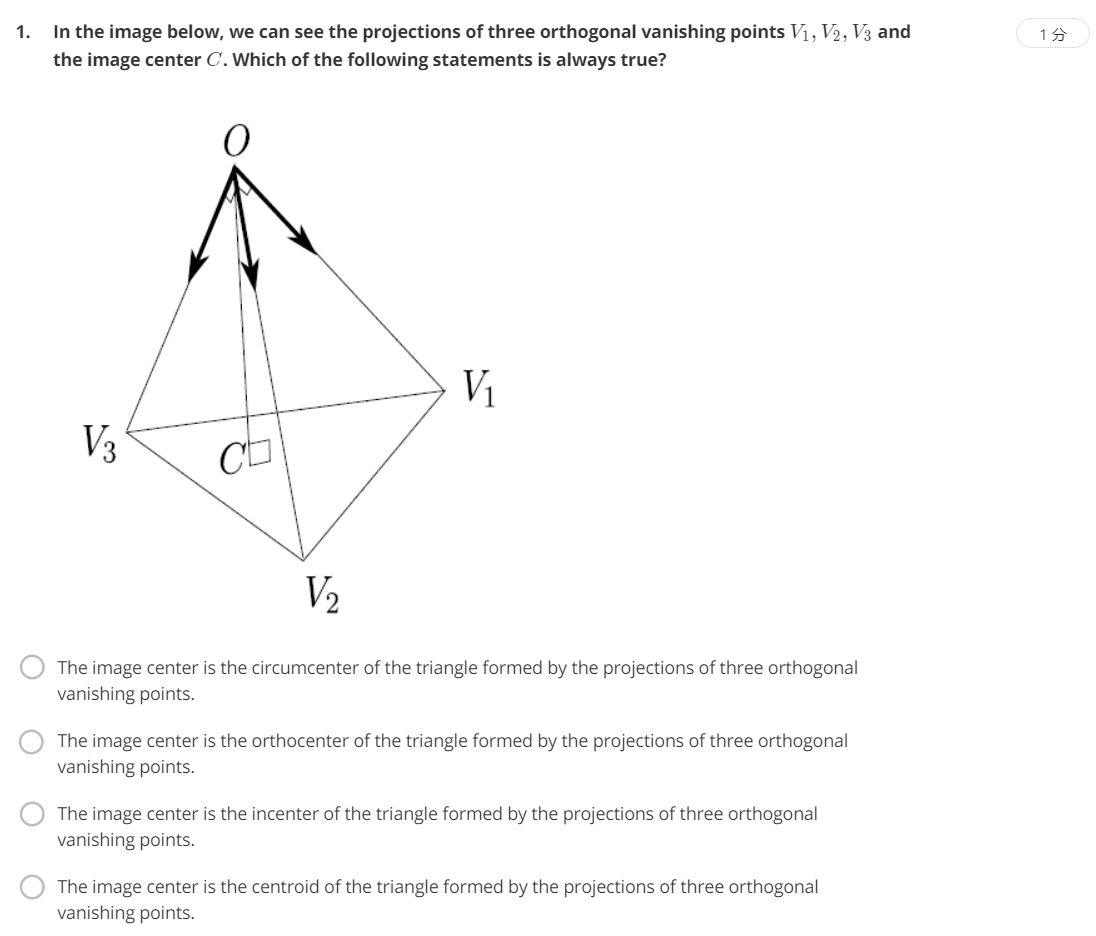C点是OC的垂足，OC是高，C点叫做三角形的垂心，选B。circumcenter是外接圆中心，orthocenter是垂心，incenter是内接圆圆心，centroid是质心。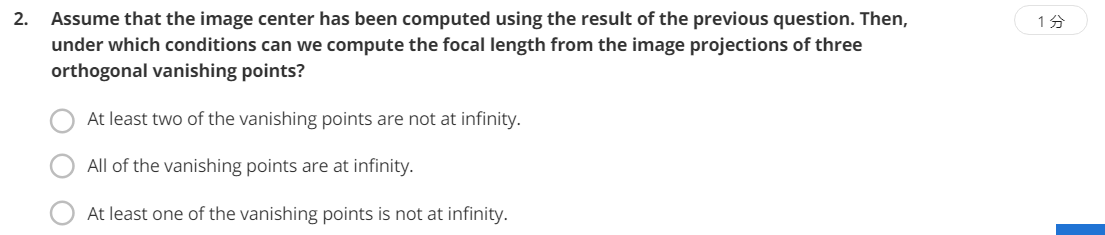还是对原理的考察，至少要有交点才可以计算。

#### 8.Camera Calibration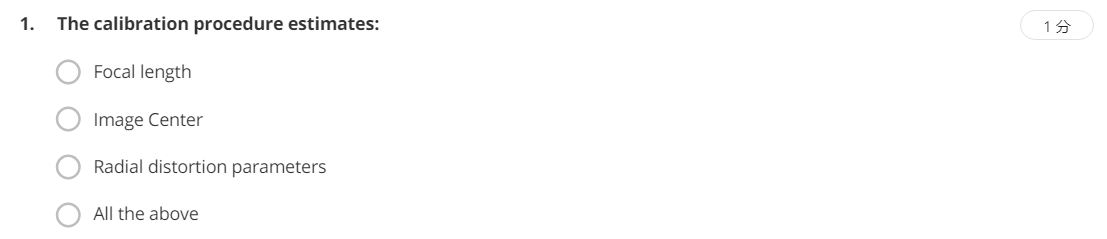标定可以获得相机的内参(焦距、主点)以及畸变参数，所以选D。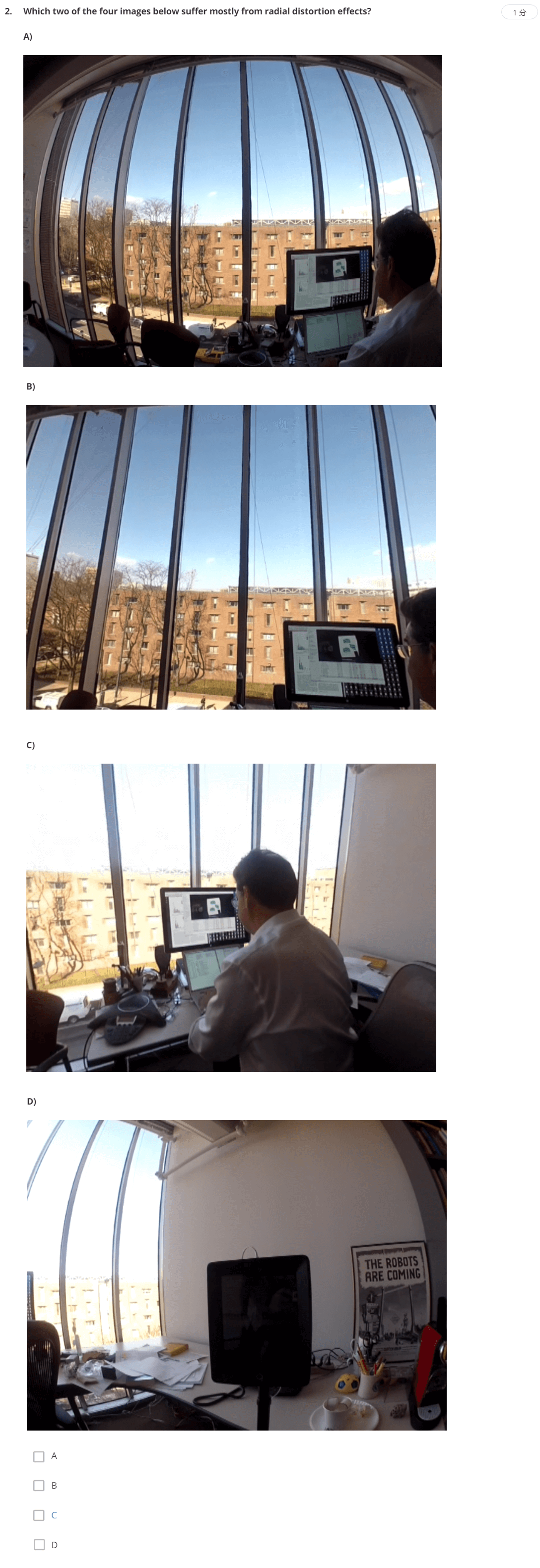题目问的是哪两张图片受径向畸变影响最大，很明显是A和D。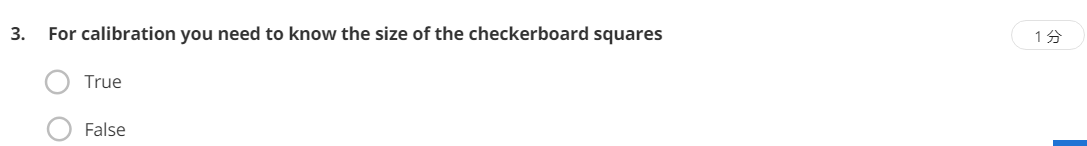在标定的过程中，需要知道标定板的尺寸(格子数)，所以是True。

#### 9.Assignment Dolly Zoom